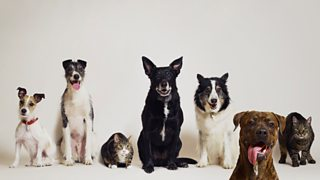# Writing a comparison in the form of a ratio

## ExampleA farmer has 2 cats and 5 dogs. Write down the ratio of cats to dogs and dogs to cats.

Cats : Dogs $$= 2 : 5$$

Dogs : Cats $$= 5 : 2$$

Now try this question:

Question

The same farmer also has 85 sheep and 15 cows. Write down the ratio of cows to sheep in its simplest form.

cows : sheep $$= 15 : 85$$

$$15$$ and $$85$$ are both divisible by 5

$\frac{{15}}{5} = 3$

$\frac{{85}}{5} = 17$

So $$15 : 85 = 3 : 17$$

A ratio is used to compare two quantities which are measured in the same units.